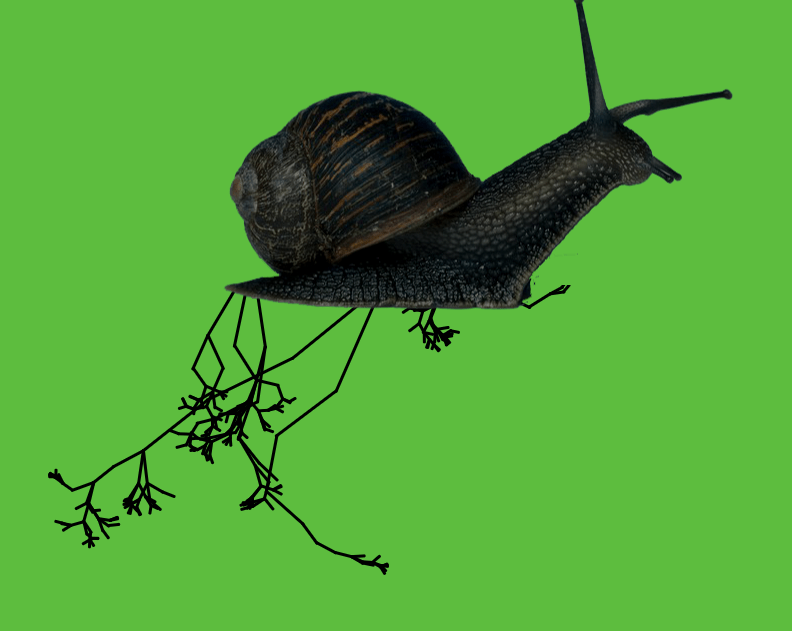# Roots and things

I’m still making generative drawing with p5.js (which I am calling “drawgramming”). This sketch generates random (and rather lovely) roots that have grown from a very slow snail. Try it out here >>> https://www.beccarose.co.uk/code/rootz/I didn’t manage to figure out how to add a button to this to generate new snail-root-growing-formations (well, I did add a button but it broke the code!). Instead you have to refresh to make new snail-root-growing-formations.

Code:
``` // using transformations // make 1 branch at a time. (so you don't have to go back and draw branches) // computer keeps track using push and pop // push forward and pop back```

//define angle
var angle;
var imgW;
var imgH;
var img;
var rootButton;

var rootleng = 100;
var rootlengLeft = 130;
var rootlenRight = 105;

println(“errr yup should be loaded”);
}

function setup() {
createCanvas(900, 700);
stroke(0);
// branchButton = createButton(‘root me up’);
//branchButton.position(40, 20);

noLoop();

imageMode(CENTER);
}

function draw() {
angle = random(PI / 16, PI / 2);

//nf(num, left unit, right unit)
println(“angle = ” + nf(angle, 1, 3));

//start from the bottom, center of the canvas (using translate as not to complicate things by using X, Y coordinates
//this will be a global translates to all function as it is in the draw and called before the function

background(19, 200, 60);
translate((width / 2)+100, height / 4);

strokeWeight(3);
//call function
push();
roots(rootleng);
translate(-rootleng, -rootleng);
rootsLeft(rootlengLeft);
translate(-rootlengLeft, -rootlengLeft);
rootsRight(rootlenRight);
// branchButton.mousePressed(branch(100));
pop();

noStroke();
fill(19, 200, 60);
rect(-250, -270, 400, 400);
tint(125);
image(img, 0, 0, imgW, imgH);
}

//this will draw an individual branch
//generation changes the thickness of line each time
function roots(length) {

//draw a single line

line(0, 0, 0, length);
// move line to end of the single line
translate(0, length);
//shrink the line
length *= random(0.4, 0.9);

//now for getting fractal
if (length > 6) {
//get a random number to make n branches
//floor function makes intergers only

var n = floor(random(1, 4));
for (var i = 0; i < n; i++) { //log where you push the whole thing push(); rotate(random(-PI / 3, PI / 3)); roots(length) //pop out of the log pop(); } } } function rootsLeft(lengthLeft) { //draw a single line //RED //stroke(200, 40, 20); line(0, 0, 0, lengthLeft); // move line to end of the single line translate(0, lengthLeft); //shrink the line lengthLeft *= random(0.4, 0.9); //now for getting fractal if (lengthLeft > 4) {

//get a random number to make n branches
//floor function makes intergers only

var n = floor(random(1, 4));
for (var i = 0; i < n; i++) { //log where you push the whole thing push(); rotate(random(-PI / 3, PI / 3)); roots(lengthLeft) //pop out of the log pop(); } } } function rootsRight(length) { //draw a single line //BLUE //stroke(49, 69, 200); line(0, 0, 0, length); // move line to end of the single line translate(0, length); //shrink the line length *= random(0.4, 0.9); //now for getting fractal if (length > 6) {

//get a random number to make n branches
//floor function makes integers only

var n = floor(random(1, 4));
for (var i = 0; i < n; i++) {
//log where you push the whole thing
push();
rotate(random(-PI / 3, PI / 3));
roots(length)
//pop out of the log
pop();
}

}
}